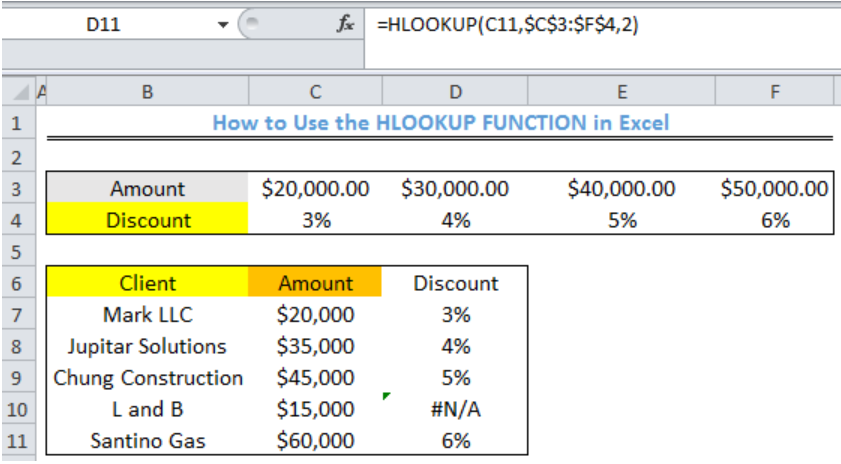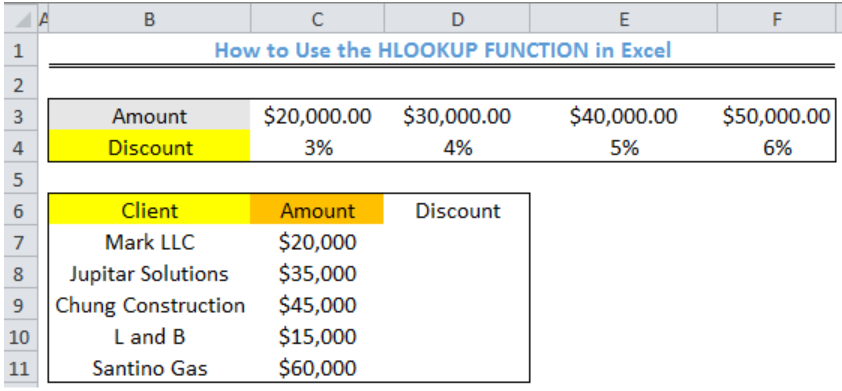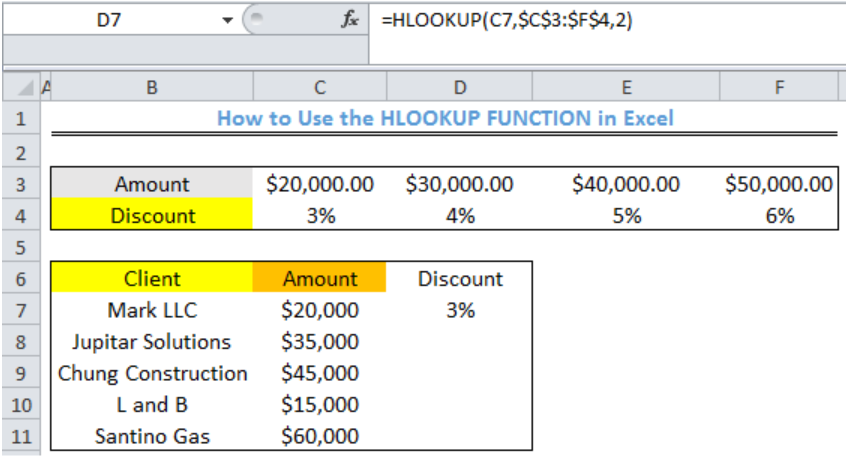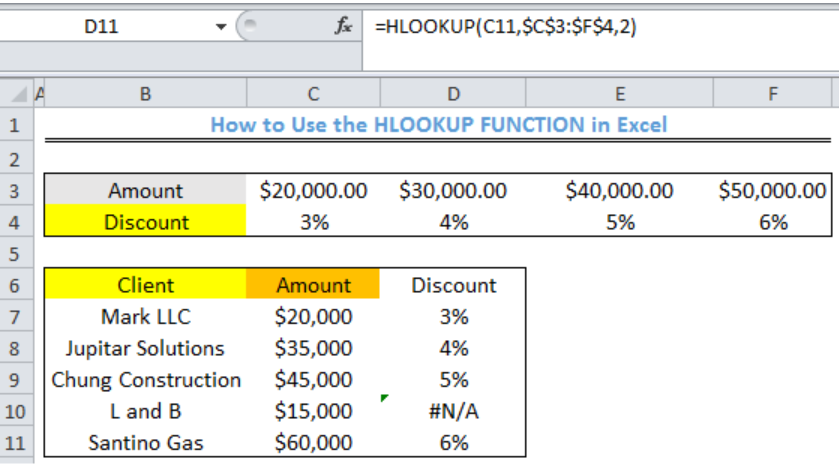Get instant live expert help with Excel or Google Sheets“My Excelchat expert helped me in less than 20 minutes, saving me what would have been 5 hours of work!”

#### Post your problem and you’ll get expert help in seconds.

Your message must be at least 40 characters
Our professional experts are available now. Your privacy is guaranteed.

# How to Use the HLOOKUP Function in Excel

We can use the HLOOKUP Function in excel to lookup and return values from a specific row in a table. “H” stands for “horizontal” in the HLOOKUP function, where lookup values appear in the first row of the table, moving horizontally to the right. Approximate and exact matching are supported by HLOOKUP and wildcards (* ?) are for finding partial matches. The steps below will walk through the process.Figure 1- How to Use the HLOOKUP Function in Excel

## Syntax

`=HLOOKUP(value,table,row_index,[range_lookup])`

• Value: This is the value to search for
• Table: This is the range to search for the lookup value
• Row_index: This number specifies the row to retrieve the data
• Range_lookup: This is used to specify if we want and approximate or exact match. If omitted, HLOOKUP assumes an approximate match

## Formula

`=HLOOKUP(C7,\$C\$3:\$F\$4,2)`

## Setting up the Data

• We will use the HLOOKUP function to find the associated discount for the amount for each client in Column A.Figure 2 – Setting up the Data

## Using the HLOOKUP Function

• We will click on Cell D7
• We will insert the formula below into Cell D7
`=HLOOKUP(C7,\$C\$3:\$F\$4,2)`
• We will press the enter keyFigure 3- HLOOKUP result for Discount for Mark LLC

• We will click on Cell D7 again
• We will double click on the fill handle tool which is the small plus sign you see at the bottom right of Cell D7. Select and drag down to copy the formula to Cell D11Figure 4- HLOOKUP results

## Note

• HLOOKUP is used when lookup values are located in the first row of a table.
• If the lookup_value is greater than every value in the lookup table, the HLOOKUP function matches the last value
• If the lookup_value is less than all values in the lookup table, the HLOOKUP function returns the #N/A error
• If we omit range_lookup, the HLOOKUP function will allow a non-exact or approximate match, but it will use an exact match if one exists. The Approximate match is set as 1 and exact match is set as 2.
• If we set the range_lookup to approximate match, then the lookup values in the first row of the table must be sorted in ascending order. Otherwise, HLOOKUP may return an incorrect or unexpected value.
• If range_lookup is FALSE (exact match), values in the first row of the table do not need to be sorted.

## Instant Connection to an Expert through our Excelchat Service

Most of the time, the problem you will need to solve will be more complex than a simple application of a formula or function. If you want to save hours of research and frustration, try our live Excelchat service! Our Excel Experts are available 24/7 to answer any Excel question you may have. We guarantee a connection within 30 seconds and a customized solution within 20 minutes.

Solution examplesHi, I have a problem with vlookup formula, I am not sure what am I doing wrong but it seems to not be working
Solved by K. F. in 40 minsI need a formula to check one cell on the 1st sheet (e2) with a list on another sheet (Sheet1) in the A column, starting at A1
Solved by O. D. in 21 minshow to make a format that if multiple cells have number contents its value is 1 in the last cell
Solved by C. C. in 28 minsI am working on scheduling rotation for a residency program. Lets say I have column â??Bâ?? titled July which has names of the rotations such as â??Cardiologyâ?, â??Nephrologyâ?, â??Floorsâ?, â??Vacationâ? etc. I have rows 1 to 65 with names of people. I want to know in a different cell who is on cardiology in July and the formula should pull in all the names. How can I do this?
Solved by I. Y. in 60 minsI need to use the answer "None" in column A to put "0" in all corresponding column B cells
Solved by X. S. in 60 mins